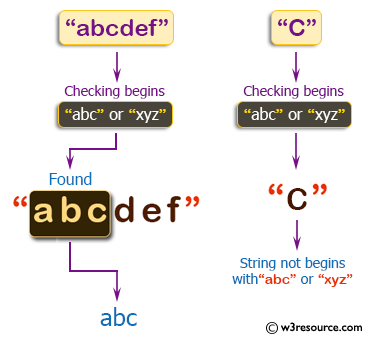﻿ C++ : Check if a given string begins with abc or xyz# C++ Exercises: Check if a given string begins with 'abc' or 'xyz'

## C++ Basic Algorithm: Exercise-74 with Solution

Write a C++ program to check if a given string begins with 'abc' or 'xyz'. If the string begins with 'abc' or 'xyz' return 'abc' or 'xyz' otherwise return the empty string.

Sample Solution:

C++ Code :

``````#include <iostream>
using namespace std;

string test(string s1)
{
if (s1.substr(0,3) == "abc")
{
return "abc";
}
else if (s1.substr(0,3) == "xyz")
{
return "xyz";
}
else
{
return "";
}
}

int main()
{
cout << test("abc") << endl;
cout << test("abcdef") << endl;
cout << test("C") << endl;
cout << test("xyz") << endl;
cout << test("xyzsder") << endl;
return 0;
}
``````

Sample Output:

```abc
abc

xyz
xyz
```

Pictorial Presentation:Flowchart:C++ Code Editor: